# GSEB Solutions Class 11 Maths Chapter 9 Sequences and Series Ex 9.3

Gujarat Board GSEB Textbook Solutions Class 11 Maths Chapter 9 Sequences and Series Ex 9.3 Textbook Questions and Answers.

## Gujarat Board Textbook Solutions Class 11 Maths Chapter 9 Sequences and Series Ex 9.3

Question 1.
Find the 20th and nth terms of the G.P., $$\frac{5}{2}$$, $$\frac{5}{4}$$, $$\frac{5}{8}$$, ……………….
Solution:
Tn = arn-1. Here a = $$\frac{5}{2}$$, r = $$\frac{5}{4}$$ + $$\frac{5}{2}$$ = $$\frac{1}{2}$$.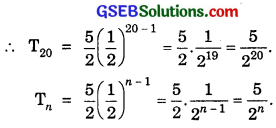Question 2.
Find the 12th term of a G.P. whose 8th term is 192 and the common ratio is 2?
Solution:
Let a be the first term and r be the common ratio of the G.P.
Then, T8 = ar8-1 = ar7 = 192 …………………… (1)
Now, T12 = ar12-1 = ar11 = (ar7)(r4)
= 192 × 24 [∵ r = 2]
= 192 × 16 = 3072.

Question 3.
The 5th, 8th and 11th terms of a G.P. are p, q and s, respectively. Show that q2 = ps.
Solution:
Let a be the first term and r be the common ratio of G.P.
Then, T5 = p ⇒ ar4 = p ………………… (1)
T8 = q ⇒ ar7 = q ………………… (2)
T11 = s ⇒ ar10 = s ………………… (3)
Now, q2 = (ar7)2 [Using (2)]
⇒ q2 = a2r14 = (ar2)(ar10)
⇒ q2 = ps [Using (1) and (3)]Question 4.
The 4th term of a G.P. is square of its 2nd term and the first term is – 3. Determine its 7th term?
Solution:
Let a be the first term and r be the common ratio of G.P. Then,
T4 of G.P. = (T2 of G.P.)2 and a = – 3.
ar3 = (ar)2
⇒ ar3 = a2r2
⇒ r = a = – 3 [∵ a = – 3, given]
Now, T7 = ar6 = (- 3)(- 3)6
= (- 3)7 = – 2187.

Question 5.
Which term of the sequence:
(a) 2, 2$$\sqrt{2}$$, 4, … is 128?
(b) $$\sqrt{3}$$, 3, 3$$\sqrt{3}$$, …………….. is 729?
(c) $$\frac{1}{3}$$, $$\frac{1}{9}$$, $$\frac{1}{27}$$, …………………., is $$\frac{1}{19683}$$?
Solution:
(a) The G.P. is 2, $$\sqrt{2}$$, 4, ……………
The first term = a = 2, the common ratio r = $$\sqrt{2}$$.
∴ nth term = arn-1 = 128.
or 2($$\sqrt{2}$$)n-1 = 128 or 2n-1/2 = 64 = 26.
⇒ $$\frac{n-1}{2}$$ = 6 or n – 1 = 12 or n = 13.

(b) Here, a = $$\sqrt{3}$$, r = $$\sqrt{3}$$.
Let Tn = 729.
⇒ arn-1 = 729
⇒ ($$\sqrt{3}$$)($$\sqrt{3}$$)n-1 = 729
⇒ ($$\sqrt{3}$$)n = (9)3
⇒ 3n/2 = (32)2 = 36
⇒ $$\frac{n}{2}$$ = 6 ⇒ n = 12.
Hence, the 12th term is 729.

(c) Here, a = $$\frac{1}{3}$$, r = $$\frac{1/9}{1/3}$$ = $$\frac{1}{9}$$ × $$\frac{3}{1}$$ = $$\frac{1}{3}$$.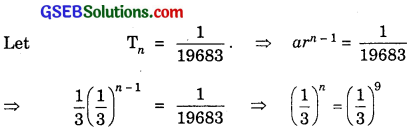⇒ n = 9.
Hence, the 9th term is $$\frac{1}{19683}$$.Question 6.
For what values of x, the numbers $$\frac{- 2}{7}$$, x, $$\frac{- 7}{2}$$ are in G.P.?
Solution:
The numbers $$\frac{- 2}{7}$$, x, $$\frac{- 7}{2}$$ will be in G.P., if
$$\frac{x}{- 2/7}$$ = $$\frac{- 7}{2/x}$$ [∵ Each = c.r.]
⇒ x2 = – $$\frac{7}{2}$$ × $$\frac{- 2}{7}$$ = 1
⇒ x = ± 1.

Find the sum to indicated number of terms of each of the following geometric progressions in questions 7 to 10:
7. 0.15, 0.015, 0.0015, ……… 20 terms.
8. $$\sqrt{7}$$, $$\sqrt{21}$$, 3$$\sqrt{7}$$, ……………… n terms.
9. 1, – a, a2, – a3, ………….. n terms (a ≠ – 1).
10. x3, x5, x7, ……………….. n terms (if x ≠ ± 1)
Solutions to questions 7 – 10:
7. Here, a = 0.15, r = $$\frac{.015}{.15}$$ = 0.1 < 1, n = 20.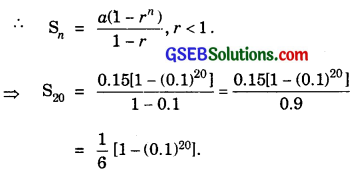8. Here, a = $$\sqrt{7}$$, r = $$\frac{\sqrt{21}}{\sqrt{7}}$$ = $$\sqrt{\frac{21}{7}}$$ = $$\sqrt{3}$$ > 1, No. of terms = n.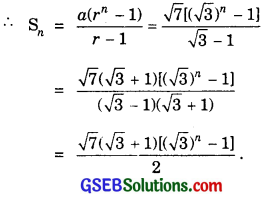9. Here a = 1, r = $$\frac{- a}{1}$$ = – a(a ≠ – 1).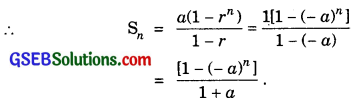10. Here a = x3, r = $$\frac{x^{5}}{x^{3}}$$ = x2, x ≠ ± 1.Question 11.
Evaluate $$\sum_{k=1}^{11}$$ (2 + 3k).
Solution:
$$\sum_{k=1}^{11}$$ (2 + 3k) = (2 + 3)1 + (2 + 3)2 + (2 + 33) + ……………. + (2 + 311)
= 2 × 11 + (31 + 32 + 33 + ………………… + 311)
= 22 + $$\frac{3\left(3^{11}-1\right)}{3-1}$$ [S = $$\frac{a\left(r^{n}-1\right)}{r-1}$$, r > 1]
= 22 + $$\frac{3}{2}$$ (311 – 1).

Question 12.
The sum of first three terms of a G.P. is $$\frac{39}{10}$$ and their product is 1. Find the common ratio and the terms?
Solution:
Let the sum of first terms of a G.P is $$\frac{a}{r}$$, a and ar, where r is the common ratio and $$\frac{a}{r}$$ is the first term.
Product of three terms = $$\frac{a}{r}$$ × a × ar = a3
∴ a3 = 1.
Sum of these terms
$$\frac{1}{r}$$ + 1 + r = $$\frac{39}{10}$$ [Putting a = 1]
Multiplying by 10r, we get
10 + 10r + 10r2 = 39r
or 10r2 + 10r – 39r + 10 = 0.
or 10r2 – 29r + 10 = 0
⇒ (2r – 5)(5r – 2) = 0
⇒ r = $$\frac{5}{2}$$ or $$\frac{2}{5}$$.
When r = $$\frac{5}{2}$$, the terms of G.P. are $$\frac{2}{5}$$, 1, $$\frac{5}{2}$$.
When r = $$\frac{2}{5}$$, the terms of G.P. are $$\frac{5}{2}$$, 1, $$\frac{2}{5}$$.Question 13.
How many terms of G.P. 3, 32, 33, ……. are needed to give the sum 120?
Solution:
Let n be the number of terms of G.P. 3, 32, 33, … to make the sum = 120.
Here a = 3 and r = 3. So, we have : S = $$\frac{a\left(a r^{n}-1\right)}{r-1}$$, r > 1
∴ Sum = $$\frac{3\left(3^{n}-1\right)}{3-1}$$ = 120
or $$\frac{3}{2}$$(3n – 1) = 120.
Multiplying both sides by $$\frac{2}{3}$$, we get
3n – 1 = 80
∴ 3n = 80 + 1 = 81 = 34.
⇒ n = 4.
∴ Required number of terms of given G.P. is 4.

Question 14.
The sum of the first three terms of G.P. is 16 and the sum of the next three terms is 128. Determine the first term, common ratio and the sum to n terms of the G.P?
Solution:
Let a be the first term and r be the common ratio.
Now, S3 = 16.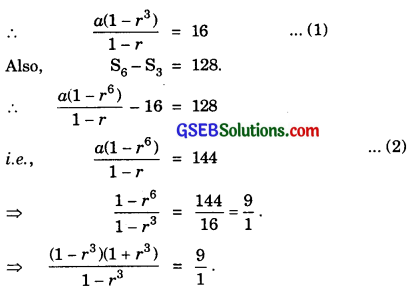⇒ 1 + r3 = 9 ⇒ r3 = 8
⇒ r3 = 23 ⇒ r = 2.
Thus, common ratio = 2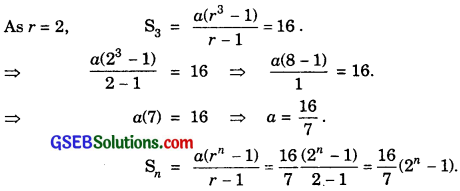Question 15.
Given a G.P. with a = 729 and 7th term 64, determine S7.
Solution:
Here, a = 729 and T7 = 64. Let c.r.
Now, T7 = 64 ⇒ ar6 = 64.
⇒ 729r6 = 64 ⇒ r6 = $$\frac{64}{729}$$.
⇒ r6 = ($$\frac{2}{3}$$)6 ⇒ r = $$\frac{2}{3}$$ < 1
⇒ Sn = $$\frac{a\left(1-r^{n}\right)}{1-r}$$.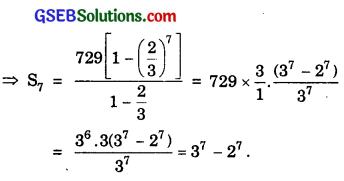= 2187 – 128 = 2059.Question 16.
Find a G.P. for which sum of the first two terms is – 4 and the fifth term is 4 times the third term?
Solution:
Let a be the first term and r the common ratio. Also,
S2 = – 4, T5 = 4T3.
⇒ $$\frac{a\left(1-r^{2}\right)}{1-r}$$ = – 4 ⇒ a(1 + r) = – 4 ……………….. (1)
Also, ar4 = 4ar2
⇒ r2 = 4 ⇒ a = $$\frac{- 4}{3}$$.
Thus, the G.P. is $$\frac{- 4}{3}$$, $$\frac{- 8}{3}$$, $$\frac{- 16}{3}$$, ………………..
When r = – 2 : a(1 – 2) = – 4 ⇒ a(- 1) = – 4 ⇒ a = 4.
Thus, the G.P. is 4, – 8, 16, – 32, 64, …

Question 17.
If the 4th, 10th and 16th terms of a G.P. are x, y and z, respectively. Prove that x, y, z are in G.P?
Solution:
Let a be the first term and r be the common ratio.
∴ T4 = x ⇒ ar3 = x ………………… (1)
T10 = y ⇒ ar9 = y ……………………. (2)
and T16 = z ⇒ ar15 = z ……………………… (3)
Now, x, y, z will be in G.P.
If ar3, ar9, ar15 are in G.P.
i.e; $$\frac{a r^{9}}{a r^{3}}$$ = $$\frac{a r^{15}}{a r^{9}}$$ ⇒ r6 = r6, which is true.
Hence x, y, z are in G.P.Question 18.
Find the sum of first n terms of the sequence 8, 88, 888, 8888, …………….
Solution:
Let S be the sum of first n terms of the sequence 8 + 88 + 888 + 8888 + ……………
∴ S = 8 + 88 + 888 + 8888 + …………….. to n terms.
= 8[1 + 11 + 111 + 1111 + …………….. to n terms]
= $$\frac{8}{9}$$ [9 + 99 + 999 + 9999 + ………….. to n terms]
= $$\frac{8}{9}$$[(10 – 1) + (102 – 1) + (103 – 1) + (104 – 1) + ……………… to n terms]
= $$\frac{8}{9}$$[10 + 102 + 103 + 104 + ……………….. to n terms] – (1 + 1 + 1 + 1 + ……………… to n terms]
= $$\frac{8}{9}$$($$\frac{10\left(10^{n}-1\right)}{10-1}-n$$)
= $$\frac{8}{9}$$[$$\frac{10}{9}$$(10n – 1) – n].

Question 19.
Find the sum of the products of the corresponding terms of the sequences 2, 4, 8, 16, 32 and 128, 32, 8, 2, $$\frac{1}{2}$$.
Solution:
Sum of products of corresponding terms of the sequences 2, 4, 8, 16, 32 and 128, 32, 8, 2, $$\frac{1}{2}$$ is:
S = 2.128 + 4.32 + 8.8 + 16.2 + 32.$$\frac{1}{2}$$, where S denotes the sum of these terms
∴ S = 256 + 128 + 64 + 32 + 16
It is a G.P., whose a = 256 and r = $$\frac{1}{2}$$. So, S = $$\frac{a\left(1-r^{5}\right)}{1-r}$$.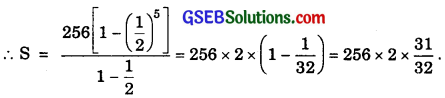= 16 × 31 = 496.Question 20.
Show that the products of the corresponding terms of the sequences a, ar, ar2, … arn-1 and A, AR, AR2, …, ARn-1 form a G.P. and find the common ratio?
Solution:
The two sequences are
a, ar, ar2, … arn-1
A, AR, AR2, … ARn-1
∴ Sum of the products of the corresponding terms of these sequences
= aA + aA(rR) + aA(r2R2) + … + aArn-1Rn-1
= aA + aA(rR) + aA(rR)2 + … + aA(rR)n-1
This is a G.P. whose first term is aA and the common ratio = aA(rR) ÷ aA = rR.

Question 21.
Find the numbers forming a geometric progression in which the third term is greater than the first term by 9, and the second term is greater than the fourth term by 18?
Solution:
Let a be the first term and r the common ratio of G.P.
Now, Tn = arn-1.
So, T2 = ar,
T3 = ar2 and T4 = ar3.
Since third term is greater than the first by 9, therefore
T3 = T1 + 9
⇒ ar2 = a + 9 ……………….. (1)
Also, the second term is greater than the fourth term by 18.
∴ T2 = T4 + 18
⇒ ar – ar3 + 18 …………….. (2)
Multiplying (1) by r, we get
ar3 = ar + 9r ……………….. (3)
From (2) and (3), we have:
ar = ar + 9r + 18.
⇒ 0 = 9r + 18 ⇒ r = $$\frac{- 18}{9}$$ = – 2.
Putting r = – 2 in (1), we get
a(- 2)2 = a + 9 ⇒ 4a = a + 9
⇒ 3a = 9 ⇒ a = 3.
Now, T2 = ar = 3(- 2) = – 6.
T3 = ar2 = 3(- 2)2 = 12
and T4 = ar3 = 3(- 2)3 = – 24.
Hence, the required terms are 3,- 6, 12 and – 24.Question 22.
If the pth, qth and rth terms of a G.P. are a, b, c respectively, prove that aq-rbr-pcp-q = 1.
Solution:
Let A be the first term and R the common ratio of G.P.
Tp = a ⇒ ARp-1 = a …………………. (1)
Tq = b ⇒ ARq-1 = b ………………. (2)
Tr = c ⇒ ARr-1 = c …………………. (3)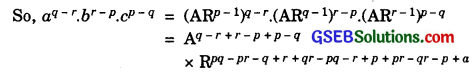= A0R0 = 1.1 = 1.

Question 23.
If the first and the nth terms of a G.P. are a and b respectively and if p is the product of first n terms, prove that p2 = (ab)n.
Solution:
Let a be the first term and r be the common ratio of G.P.
Here, T1 = a and Tn = b.
So, arn-1 = b ………………. (1)
Also, p = T1.T2.T3………..Tn
= a.ar.ar2……….arn-1
= an.r1+2+3+…………+(n – 1)
Also, 1 + 2 + 3 + ……………. + (n – 1) = $$\frac{(n – 1)n}{2}$$
= an.$$r^{\frac{n(n-1)}{2}}$$
⇒ p2 = a2n.r(n-1)n = [a2rn-1]n = [a.arnr]n
= (a.b)n [Using (1)]
Hence, p2 = (ab)n.Question 24.
Show that the ratio of the sum of first n terms of a G.P. to the sum of terms from (n + 1)th to (2n)th terms is $$\frac{1}{r^{n}}$$?
Solution:
Sum of first n terms of a G.P.
a + ar + ar2 + …………….. + arn-1
= $$\frac{a\left(1-r^{n}\right)}{1-r}$$ ……………….. (1)
∴ Sum of terms from (n + 1)th to (2n)th terms
= Tn+1 + Tn+2 + Tn+3 + …………….. + T2n
= arn + arn+1 + arn+2 + ………………. + ar2n-1
No. of terms = n.
Dividing (1) by (2), we get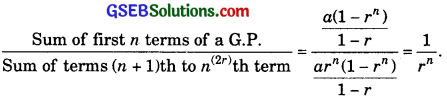Question 25.
If a, b, c are in G.P., show that
(a2 + b2 + c2)(b2 + c2 + d2) = (ab +bc + cd)2.
Solution:
Let r be the common ratio of the G.P. a, b, c, d.
Then, b = ar, c = ar2 and d = ar3.
L.H.S. = (a2 + b2 + c2)(b2 + c2 + d2)
= (a2 + a2r2 + a2r4)(a2r2 + a2r4 + a2r6)
= a4r2(1 + r2 + r4)2
and R.H.S. = (ab + bc + cd)2
= (a2r + a2r3 + a2r5)2
= a4r2(1 + r2 + r4)2.
Hence, (a2 + b2 + c2)(b2 + c2 + d2) = (ab + bc + cd)2.Question 26.
Insert two numbers between 3 and 81 so that the resulting sequence is G.P.
Solution:
Let G1, G2 be the two numbers such that 3, G1, G2, 81 are in G.P.
Let r be the common ratio.
T4 = ar4-1 = 81 or 3.r3 = 81.
∴ r3 = $$\frac{81}{3}$$ = 27 = 33 ⇒ r = 3
∴ G1 = ar = 3.3 = 9
and G2 = ar2 = 3.32 = 27.
∴ 9 and 27 are the required numbers.

Question 27.
Find the value of n so that $$\frac{a^{n+1}+b^{n+1}}{a^{n}+b^{n}}$$ may be that geometric mean between a and b?
Solution:
The G.M. between a and b = $$\sqrt{ab}$$.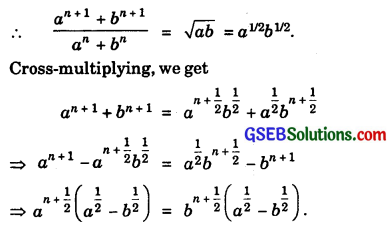Since a and b are different, cancelling a1/2 – b1/2 from both sides, we get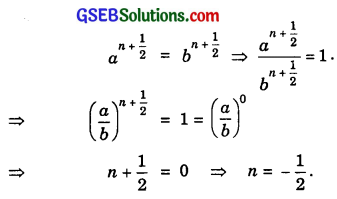Question 28.
The sum of two numbers is 6 times their geometric mean. Show that the numbers are in the ratio 3 + 2$$\sqrt{2}$$: 3 – 2$$\sqrt{2}$$.
Solution:
Let numbers be a and b.
As per problem:
Sum of two numbers = 6 × their G.M.
⇒ a + b = 6$$\sqrt{ab}$$
⇒ $$\frac{a+b}{2 \sqrt{a b}}$$ = $$\frac{3}{1}$$.
Applying componendo and dividendo, we have: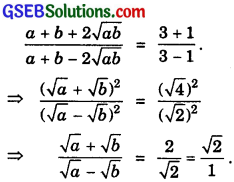Again applying componendo and dividendo, we get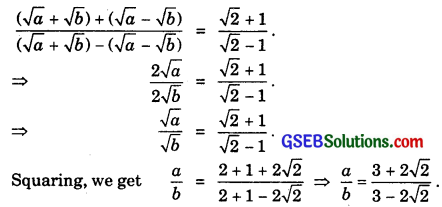Hence, the numbers are in the ratio 3 + 2$$\sqrt{2}$$ : 3 – 2$$\sqrt{2}$$.

Question 29.
If A and G be A.M. and G.M. respectively between two positive numbers, prove that the numbers are A ± $$\sqrt{(A + G)(A – G)}$$.
Solution:
Let a and b be the two numbers.
∴ A = $$\frac{a+b}{2}$$ ……………….. (1)
and G = $$\sqrt{ab}$$
or G2 = ab ………………… (2)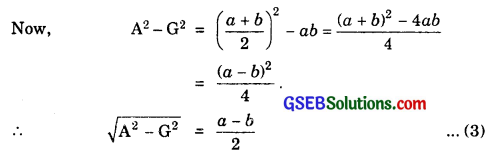Also, A = $$\frac{a+b}{2}$$ ……………………….. (4)
Adding (3) and (4), we get
A + $$\sqrt{\mathrm{A}^{2}-\mathrm{G}^{2}}$$ = a.
Subtracting (3) from (4), we get
A – $$\sqrt{\mathrm{A}^{2}-\mathrm{G}^{2}}$$ = $$\frac{a+b}{2}$$ – $$\frac{a-b}{2}$$ = b.
Then, A ± $$\sqrt{(A + G)(A – G)}$$ are the required numbers.Question 30.
The number of bacteria in a certain culture doubles every hour. If there were 30 bacteria present in the culture originally, how many bacteria will be present at the end of 2nd hour, 4th hour and nth hour?
Solution:
Number of bacteria present in the culture form a G.P.,
whose first term a = 30 and common ratio r = 2.
∴ Bacteria present after 2 hours = ar2 = 30 × 22 = 30 × 4 = 120.
Bacteria present after 4 hours = ar4 = 30 × 24 = 30 × 16 = 480.
Bacteria present after n hours = arn = 30 × 2n = 30.2n.

Question 31.
What will? ₹ 500 amounts to in 10 years after its deposit in a bank which pays annual interest of 10% compounded annually?
Solution:
Let A, P, r and T be the amount, principal, rate of interest per cent per annum and period in years respectively. The amount A is given by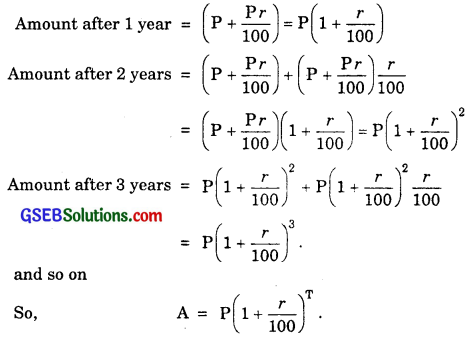Here, P = ₹ 500, r = 10% p.a.
and T = 10 years
∴ A = 500(1 + $$\frac{10}{100}$$)10 = 500 × (1.1)10Question 32.
If A.M. and G.M. of roots of a quadratic equation are 8 and 5 respectively, then obtain the quadratic equation?
Solution:
Let α and β be the roots of the quadratic equation.
∴ A.M. of α and β = $$\frac{α+β}{2}$$ = 8
and G.M. of α and β = αβ = 52
∴ α + β = 16 and αβ = 25.
Equation whose roots are α and β is
x2 – (α + β)x + αβ = 0
x2 – 16x + 25 = 0.